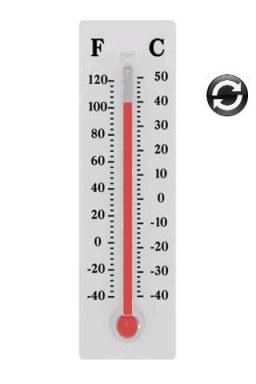# At 5:00

At 5:00 AM, the room temperature was 20°C. After six hours, the reading was 28°C. The temperature rose to how many degrees? What was the average change in temperature per hour?

Δ =  8 °C
x =  1.3333 °C/h

### Step-by-step explanation:Did you find an error or inaccuracy? Feel free to write us. Thank you!

Tips for related online calculators
Need help calculating sum, simplifying, or multiplying fractions? Try our fraction calculator.
Do you want to convert time units like minutes to seconds?